# RBSE Maths Class 8 Chapter 9: Important Questions and Solutions

RBSE Maths Chapter 9 – Algebraic Expressions Mathematics Class 8 Important questions and solutions are available here. The additional, exercise important questions and solutions of Chapter 9, available at BYJU’S, contain detailed explanations. All these important questions are based on the new pattern designed by the RBSE. Students can also get the syllabus and textbooks on RBSE Class 8 solutions.

Chapter 9 of the RBSE Class 8 Maths will help the students to solve problems related to power of expression, like and unlike terms, addition and subtraction of algebraic expressions, multiplication of algebraic expressions, multiplying a monomial by a monomial, multiplying a binomial or a trinomial by a monomial, multiplying a binomial by a binomial, multiplying a binomial by trinomial, standard identities, application of identities.

### RBSE Maths Chapter 9: Exercise 9.1 Textbook Important Questions and Solutions

Question 1: Find the product of the following pairs of monomials.

(i) 3, 5x

(ii) -5p, -2q

(iii) 7t2, -3n2

(iv) 6m, 3n

(v) -5x2, -2x

Solution:

[i] 3 * (5x)

= 3 * 5 * (x)

= 15x

[ii] -5p, -2q

= (-5) x p x (-2) x q

= (-5) x (-2) x pq

= 10pq

[iii] 7t2, -3n2

= 7 x t2 x (-3) x n2

= 7 x (-3) x t2 x n2

= -21t2n2

[iv] 6m, 3n

= 6 x m x 3 x n

= 6 x 3 x m x n

= 18 mn

[v] -5x2, -2x

= (-5) * (x2) * (-2) * (-x)

= (-5) * (-2) * (x2) * (-x)

= 10x3

Question 2: Complete the table below.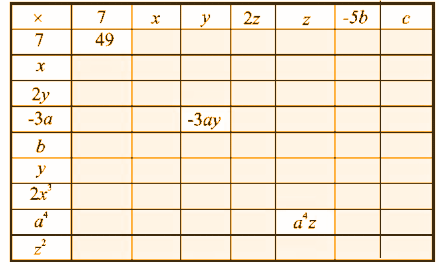Solution:

 * 7 x y 2z a -5b c 7 49 7x 7y 14z 7a -35b 7c x 7x x2 xy 2zx ax -5bx cx 2y 14y 2xy 2y2 4yz 2ay -10by 2cy -3a -21a -3ax -3ay -6az -3a2 15ab -3ac b 7b bx by 2bz ab -5b2 bc y 7y xy y2 2yz ay -5by cy 2x3 14x3 2x4 2x3y 4x3z 2x3a -10x3b 2x3c a4 7a4 a4x a4y 2a4z a5 -5a4b a4c z2 7z2 xz2 yz2 2z3 az2 -5bz2 cz2

Question 3: Multiply the following monomial.

[i] xy, x2y, xy, x

[ii] m, n, mn, m3n, mn3

[iii] kl, lm, km, klm

Solution:

[i] xy, x2y, xy, x

= (x) * (y) * (x) * (x) * (y) * (x) * (y) * (x)

= (x) * (x) * (x) * (x) * (x) * (y) * (y) * (y)

= x5y3

[ii] m, n, mn, m3n, mn3

= m x n x m x n x m x m x m x n x m x n x n x n

= m x m x m x m x m x m x n x n x n x n x n x n

= m6n6

[iii] kl, lm, km, klm

= k x l x l x m x k x m x k x l x m

= k x k x k x l x l x l x m x m x m

= k3l3m3

Question 4: Find the simple interest = [PTR] / , if principal P = 4x2, time (T) = 5x and rate of interest R = 5y.

Solution:

Simple interest = [PTR] / 

= [(4x2) * (5x) * (5y)] / 

= [(4 * (x) * (x) * 5 * (x) * 5 * (y)] / 

= [4 * 5 * 5 * (x) * (x) * (x) * (y)] / 

= [100x3y] / 

= x3y

### RBSE Maths Chapter 9: Exercise 9.2 Textbook Important Questions and Solutions

Question 1: Multiply the binomials given below.

[i] (2x + 5) and (3x – 7)

[ii] (x – 8) and (3y + 5)

[iii] (1.5p – 0.5q) and (1.5p + 0.5q)

[iv] (a + 3b) and (x + 5)

[v] (2lm + 3l2) and (3lm – 5l2)

[vi] [(¾)a2 + 3b2] and [4a2 – (5/3)b2]

Solution:

[i] (2x + 5) and (3x – 7)

= 2x (3x – 7) + 5 (3x – 7)

= (2x) * (3x) – (2x) * 7 + 5 * (3x) – (5 * 7)

= 2 * (x) * 3 * (x) – 2 * (x) * 7 + 5 * 3 * (x) – 35

= 2 * 3 * (x) * (x) – 2 * 7 * (x) + 15 * (x) – 35

= 6 * (x2) – 14 * (x) + 15x – 35

= 6x2 – 14x + 15x – 35

= 6x2 + x – 35

[ii] (x – 8) and (3y + 5)

= x (3y + 5) – 8 (3y + 5)

= (x) * 3y + (x) * 5 – 8 * 3 * y – 8 * 5

= (x) * 3y + 5x – 8 * 3 * y – 40

= 3 * (x) * y + 5x – 24 * y – 40

= (3x) * y + 5x – 24y – 40

= 3xy + 5x – 24y – 40

[iii] (1.5p – 0.5q) and (1.5p + 0.5q)

= 1.5p (1.5p + 0.5q) – 0.5q (1.5p + 0.5q)

= 1.5p x 1.5p + 1.5p x 0.5q – 0.5q x 1.5p – 0.5q x 0.5q

= 2.25p2 x + 0.75 x pq – 0.75 x qp – 0.25 q2

= 2.25p2 + 0.75pq – 0.75pq – 0.25q2

= 2.25p2 – 0.25q2

[iv] (a + 3b) and (x + 5)

= a (x + 5) + 3b (x + 5)

= a x (x) + a x 5 + 3b x (x) + 3b x 5

= ax + 5a + 3bx + 3 x 5 x b

= ax + 5a+ 3bx + 15b

[v] (2lm + 3l2) and (3lm – 5l2)

= 2lm (3lm – 5l2) + 3l2 (3lm – 5l2)

= [2lm x 3lm] – [2lm x 5l2] + 3l2 x 3lm – [3l2 x 5l2]

= 6l2m2 – 10l3m + 9l3m – 15l4

= 6l2m2 – l3m – 15l4

[vi] [(¾)a2 + 3b2] and [4a2 – (5/3)b2]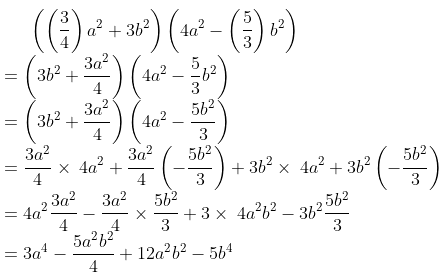Question 2: Find the product of the following.

[i] (3x + 8) (5 – 2x)

[ii] (x + 3y) (3x – y)

[iii] (a2 + b) (a + b2)

[iv] (p2 – q2) (2p + q)

Solution: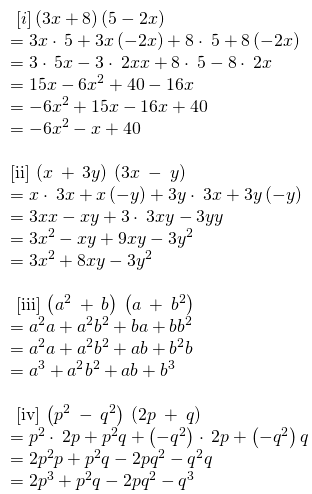Question 3: Simplify the following.

[i] (x + 5) (x – 7) + 35

[ii] (a2 – 3) (b2 + 3) + 5

[iii] (t + s2) (t2 – s)

[iv] (a + b) (c – d) + (a – b) (c + d) + 2 (ac + bd)

[v] (a + b) (a2 – ab + b2)

[vi] (a + b + c) (a + b – c)

[vii] (a + b) (a – b) – a2 + b2

Solution: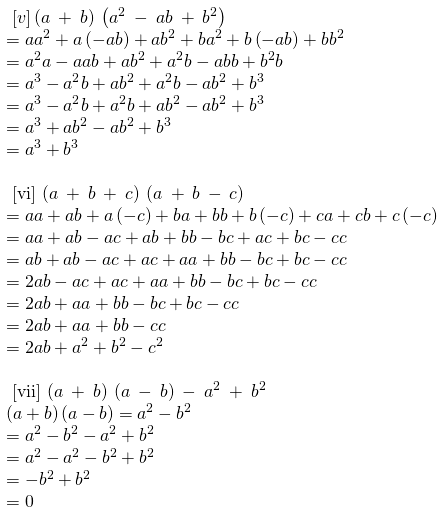### RBSE Maths Chapter 9: Exercise 9.3 Textbook Important Questions and Solutions

Question 1: Find the products using a suitable identity.

[i] (x + 5) (x + 5)

[ii] (3x + 2) (3x + 2)

[iii] (5a – 7) (5a – 7)

[iv] (3p – [½]) (3p – [½])

[v] (1.2m – 0.3) (1.2m + 0.3)

[vi] (x2 + y2) (x2 – y2)

[vii] (7a – 9b) (7a – 9b)

Solution: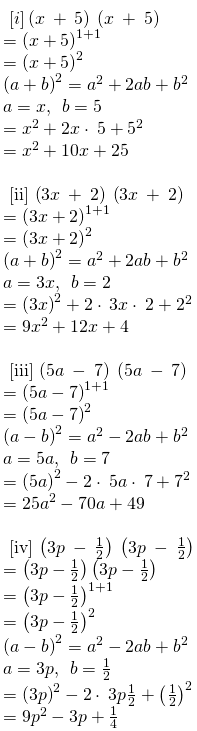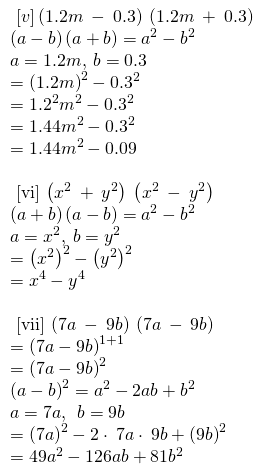Question 2: Use the identity (x + a) (x + b) = x2 + (a + b)x + ab to find the following products.

[i] (x + 1) (x + 2)

[ii] (3x + 5) (3x + 1)

[iii] (4x – 5) (4x – 1)

Solution:

[i] (x + 1) (x + 2)

Using the identity (x + a) (x + b) = x2 + (a + b)x + ab

a = 1; b = 2

= x2 + (1 + 2)x + 2 x 1

= x2 + 3x + 2

[ii] (3x + 5) (3x + 1)

Using the identity (x + a) (x + b) = x2 + (a + b)x + ab

a = 5; b = 1

= [3x]2 + (5 + 1)* 3x + 5 x 1

= 9x2 + 6 * 3x + 5

= 9x2 + 18x + 5

[iii] (4x – 5) (4x – 1)

Using the identity (x + a) (x + b) = x2 + (a + b)x + ab

a = (-5); b = (-1)

= [4x]2 + ([-5] + [-1]) * 4x + (-5 * -1)

= 16x2 + (-6) * 4x + 

= 16x2 – 24x + 5

Question 3: Find the following squares by using identities.

[i] (b – 7)2

[ii] (xy + 3z)2

Solution: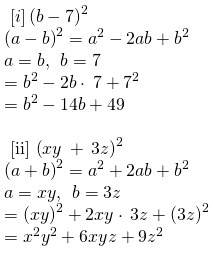Question 4: Simplify the following problems.

[i] (a2 – b2)2

[ii] (2n + 5)2 – (2n – 5)2

Solution: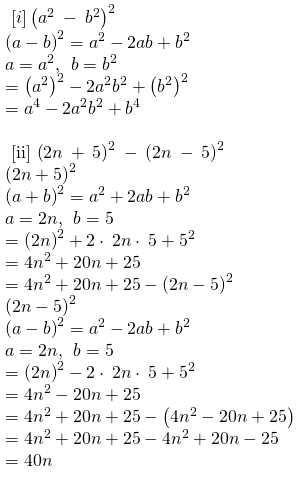Question 5: Show that

[i] (2a + 3b)2 – (2a – 3b)2 = 24ab

[ii] (4x + 5)2 – 80x = (4x – 5)2

Solution: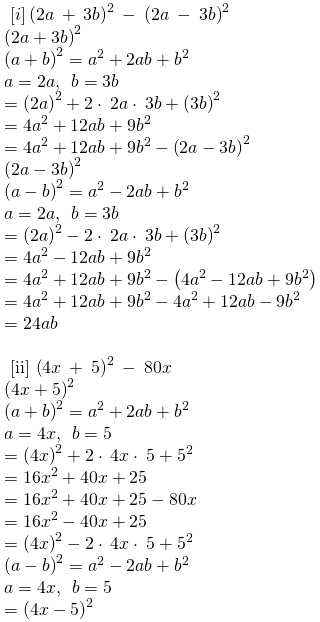Question 6: Using the identities, evaluate the following.

[i] 992

[ii] 1032

Solution:

[i] 992

= (100 – 1)2

(a – b)2 = a2 – 2ab + b2

a = 100; b = 1

= (100)2 – 2 * (100) * (1) + 12

= 10000 – 200 + 1

= 9801

[ii] 1032

= (100 + 3)2

(a + b)2 = a2 + 2ab + b2

a = 100; b = 3

= (100)2 + 2 * (100) * (3) + 32

= 10000 + 600 + 9

= 10609

Question 7: Using a2 – b2 = (a + b) (a – b), find the values of the following.

[i] 102 – 992

[ii] (10.3)2 – (9.7)2

Solution:

[i] 102 – 992

= (10 + 99) (10 – 99)

= (109) (-89)

= -9701

[ii] (10.3)2 – (9.7)2

= (10.3 + 9.7) (10.3 – 9.7)

= (20) (0.6)

= 12

Question 8: Using a2 – b2 = (a + b) (a – b), find the values of the following.

[i] 103 x 102

[ii] 7.1 x 7.3

[iii] 102 x 99

[iv] 9.8 x 9.6

Solution:

[i] 103 x 102

= (100 + 3) (100 + 2)

Using the identity (x + a) (x + b) = x2 + (a + b)x + ab

a = 3; b = 2

= 1002 + (3 + 2) * 100 + [3 x 2]

= 10000 + 5 * 100 + 6

= 10000 + 500 + 6

= 10506

[ii] 7.1 x 7.3

= (7 + 0.1) (7 + 0.3)

Using the identity (x + a) (x + b) = x2 + (a + b)x + ab

a = 0.1; b = 0.3

= 72 + [0.1 + 0.3] * 7 + [0.1 x 0.3]

= 49 + [0.4] * 7 + 0.03

= 49 + 2.8 + 0.03

= 51.83

[iii] 102 x 99

= (100 + 2) (100 – 1)

Using the identity (x + a) (x + b) = x2 + (a + b)x + ab

a = 2; b = (-1)

= 1002 + (2 + [-1]) * 100 + (2) * (-1)

= 10000 + 100 – 2

= 10098

[iv] 9.8 x 9.6

= (9 + 0.8) (9 + 0.6)

Using the identity (x + a) (x + b) = x2 + (a + b)x + ab

a = 0.8; b = 0.6

= 92 + [0.8 + 0.6] * 9 + [0.8 x 0.6]

= 81 + [1.4] * 9 + 0.48

= 81 + 12.6 + 0.48

= 94.08